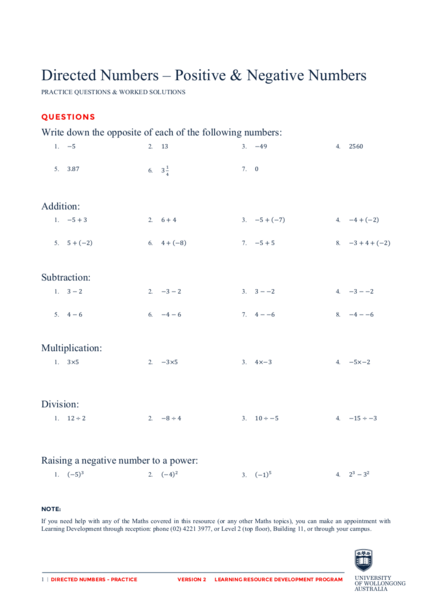# Directed Numbers: Multiplication and Division WorksheetContributed by:Directed numbers: multiplication and division worksheet. In multiplication, the numbers being multiplied are called factors; the result of the multiplication is called the product. In division, the number being divided is the dividend, the number that divides it is the divisor, and the result of the division is the quotient.
1. Directed Numbers – Positive & Negative Numbers
PRACTICE QUESTIONS & WORKED SOLUTIONS
Write down the opposite of each of the following numbers:
1. −5 2. 13 3. −49 4. 2560
5. 3.87 ! 7. 0
6. 3
!
1. −5 + 3 2. 6+4 3. −5 + −7 4. −4 + −2
5. 5 + −2 6. 4 + −8 7. −5 + 5 8. −3 + 4 + (−2)
1. 3−2 2. −3 − 2 3. 3 − −2 4. −3 − −2
5. 4−6 6. −4 − 6 7. 4 − −6 8. −4 − −6
1. 3×5 2. −3×5 3. 4×−3 4. −5×−2
1. 12 ÷ 2 2. −8 ÷ 4 3. 10 ÷ −5 4. −15 ÷ −3
Raising a negative number to a power:
! !
1. −5 2. −4 3. −1 ! 4. 2! − 3!
If you need help with any of the Maths covered in this resource (or any other Maths topics), you can make an appointment with
Learning Development through reception: phone (02) 4221 3977, or Level 2 (top floor), Building 11, or through your campus.
1 | DIRECTED NUMBERS - PRACTICE VERSION 2 LEARNING RESOURCE DEVELOPMENT PROGRAM
2. W ORKED SOLUTIONS
Writing the opposites:
1. 5 2. −13 3. 49
4. −2560 5. −3.87 !
6. −3
!
7. 0 (the only number that is its own opposite)
1. −2 (you deposit 3 but your debt has not been cleared hence a negative balance)
2. 10 (straightforward)
3. −12 (you are going further into debt)
4. −6 (you are going further into debt)
5. 3 (you are withdrawing 2 from a balance of 5)
6. −4 (you are withdrawing 8 from a balance of only 4)
7. 0 (you are clearing your debt)
8. −3 + 4 gives 1, then 1 + −2 gives − 1
1. 1 (straightforward)
2. −5 (you are going further into debt)
3. 5 (two negatives cancel out)
4. −1 (we would get −3 + 2)
5. −2 (you are going into debt again)
6. −10 (you are going further into debt!)
7. 10 (the withdrawal has been cancelled, you are better off by 6)
8. 2 (the withdrawal has been cancelled, you are better off by 6)
1. 15 (straightforward)
2. −15 (5 withdrawals of \$3 each)
3. −12 (4 withdrawals of \$3 each)
4. 10 (5 cancellations of \$2 withdrawals or vice versa)
1. 6 (straightforward)
2. −2 (does not make sense without thinking about cancellations)
3. −2 (does not make sense without thinking about cancellations)
4. 5 (how many withdrawals of \$3 made a debt of \$15?)
Raising a negative number to a power:
1. −125 2. 16
3. −1 4. 8 − 9 = −1
2 | [RESOURCE TITLE] VERSION # LEARNING RESOURCE DEVELOPMENT PROGRAM